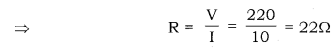# Calculate the resistance of an electric bulb which allows a 10A current when connected to a 220V power source

Calculate the resistance of an electric bulb which allows a 10A current when connected to a 220V power source.

We have,
Current flowing through the electric bulb, I = 10A
Voltage of the source in the circuit, V = 220V
According to Ohm’s Law, V = IR
Where, R is the resistance of the electric bulbTherefore, the resistance of the electric bulb is 22Ω.